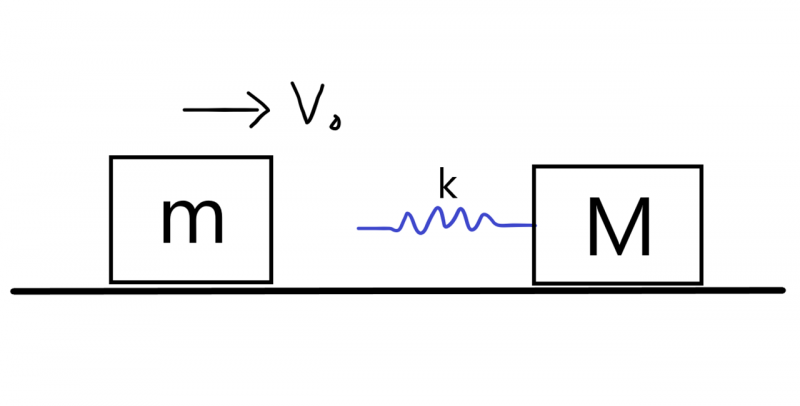# 碰撞 X 雙振子(一)：自己用python模擬！$m$ 的速度 $v_1$，為$x_1$的一次微分，如果$x_1$的一次微分微出來是負的，就代表此時速度向左；$x_1$ 的一次微分微出來是正的，就代表此時速度向右。同樣的，若二次微分微出來是負的，代表加速度向左；二次微分微出來是正的，就代表此時速度向右。$M$ 也是如此道理，就不再贅述。

#作者: Grandy  日期:2021/8/3
#雙振子碰撞時間模擬
dt=0.0000001
m=5
M=10
Vm,VM=50,0   #初速
am,aM=0,0   #初加速度
XM,Xm=0,0
k=8

i=0     #記數，算經過多少time=i*t
while (XM-Xm)<=0:
am=k*(XM-Xm)/m
aM=k*(Xm-XM)/M
Vm=Vm+am*dt
VM=VM+aM*dt
Xm=Xm+Vm*dt
XM=XM+VM*dt
i+=1
t=i*dt
print(t)Grandy